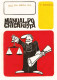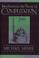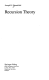### Mathematical Logic and Computability

#### Course Description

The course is divided into three parts. In the first part we consider propositional logic. The main aim of the first part is to emphasize the difference between syntax and semantics. The first part is a motivation and introduction for the second part in that we study first-order logic. Specially considered is the undecidability of first-order logic, and a necessity of a formal definition of algorithm is emphasized. In the third part we study theory of computation. RAM-machines are considered as a basic model of computation. Then we define the class of partial recursive function, and prove that the class of partial recursive functions is equal to the class of RAM-computable functions. It is a motivation for Church thesis.

#### General Competencies

Basics of classical propositional logic, first-order logic and modal logic. Fundamental knowledge on computability and recursion theory. Experience in determination of normal forms, applying semantic tableux, and determination of recursivness of functions and sets.

#### Learning Outcomes

1. convert a formula of propositional logic to normal form
2. define a notion of proof and theorem
3. practice semantic tableux
4. reproduce the basic facts on modal logic
5. define a notion of first-order structure
6. convert a first-order formula to prenex normal form
7. define the notions of RAM-computable and partial recursive functions
8. classify the import of Church thesis

#### Forms of Teaching

Lectures

Lectures which contain large number of examples and problems.

Exams

Mid-term and final exam during the lectures-free weeks.

Consultations

At least once a week.

Other

Home-works.

#### Grading Method

Continuous Assessment Exam
Type Threshold Percent of Grade Threshold Percent of Grade
Homeworks 0 % 10 % 0 % 10 %
Mid Term Exam: Written 0 % 45 % 0 %
Final Exam: Written 0 % 45 %
Exam: Written 0 % 90 %

#### Week by Week Schedule

1. The basic on set theory. Introduction to propositional logic. Syntax of propositional logic. Semantics of propositional logic.
2. Normal forms in propositional logic. Semantic tableux. Decidability. Problem P=NP.
3. Axiomatic system for propositional logic. Proof, theorem and deduction. Theorem of adequacy. Consistency.
4. A sketch of proof of completeness theorem for propositional logic.
5. Modal logic: system K, Kripke frame and model, theorems on completeness and adequacy of system K.
6. First-order logic: syntax of a first-order theory, terms and formulas.
7. Semantics of first order logic: interpretation, truth of formulas. Prenex normal form.
8. Midterm exam.
9. Semantic tableux for first-order logic. Church theorem.
10. Axiomatic system for first-order logic. Proof, theorem and deduction. Theorem of deduction. Theorem od adequacy.
11. Consistency. A sketch of proof of Goedel completeness theorem. Compactness theorem.
12. Computability: RAM-machine, RAM-computable function. Macro-machine.
13. Primitive recursive functions: initial functions, composition, primitive recursion. Ackermann function. Partial recursive function. Recursive sets.
14. Proof that each partial recursive function is RAM-computable. Coding of finite sequence of natural numbers. Coding of RAM-machine. Universal function.
15. Kleene normal form theorem on partial recursive function. The equality of classes of RAM-computable function and partial recursive functions. Recursion theorem. Rice theorem. Church thesis. Halting problem. A sketch oof proof of Church theorem.

#### Study Programmes

##### University graduate
Computer Engineering (profile)
Mathematics and Science (1. semester)
Computer Science (profile)
Mathematics and Science (1. semester)
Control Engineering and Automation (profile)
Mathematics and Science (1. semester)
Electrical Engineering Systems and Technologies (profile)
Mathematics and Science (1. semester)
Electrical Power Engineering (profile)
Mathematics and Science (1. semester)
Electronic and Computer Engineering (profile)
Mathematics and Science (1. semester)
Electronics (profile)
Mathematics and Science (1. semester)
Information Processing (profile)
Mathematics and Science (1. semester)
Software Engineering and Information Systems (profile)
Mathematics and Science (1. semester)
Telecommunication and Informatics (profile)
Mathematics and Science (1. semester)
Wireless Technologies (profile)
Mathematics and Science (1. semester)

#### Literature

Mladen Vuković (2009.), Matematička logika, Element, Zagreb
Mladen Vuković (2007.), Izračunljivost, web-izdanjeR. Cori, D. Lascar (2000.), Mathematical Logic, Part I, Oxford University PressM. Sipser (1997.), Introduction to the Theory of Computation, PWS Pub. Company;1997.J. R. Shoefield (1993.), Recursion Theory, Springer-Verlag

#### General

ID 61648
Winter semester
4 ECTS
L0 English Level
L1 e-Learning
45 Lectures
0 Exercises
0 Laboratory exercises
0 Project laboratory

85 Excellent
75 Very Good
60 Good
45 Acceptable